# VALUE Function

Converts a text string that represents a number into a number

## What is the VALUE Function?

The VALUE Function is categorized under Excel Text functions. It will convert a text string that represents a number into a number. Thus, the function will convert text that appears in a recognized format (a number, date, or time format) into a numeric value.

In financial analysis, the VALUE function is not used much, as Excel automatically converts text to numeric values. However, it is important for anyone who wants to master the full extent of Excel functions.

### Formula

=VALUE(Text)

1. Text (required argument) – This is the text enclosed in quotation marks or a reference to a cell containing the text you want to convert.

### How to use the VALUE Function in Excel?

The VALUE function was introduced in Excel 2007 and is available in all subsequent Excel versions. To understand the uses of the function, let us consider a few examples:

#### Example 1

Let’s see how this function will behave when we give the following formulas: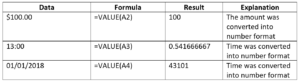We get the results below: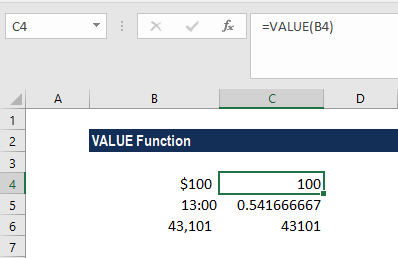#### Example 2

Suppose an HR team wishes to assign a penalty point for an employee who arrives late. For assigning penalty points based on an amount of time tardy, you can use a nested IF formula.

Suppose we are given the data below: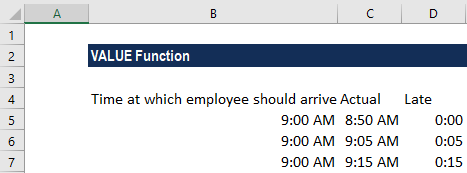The formula to use is: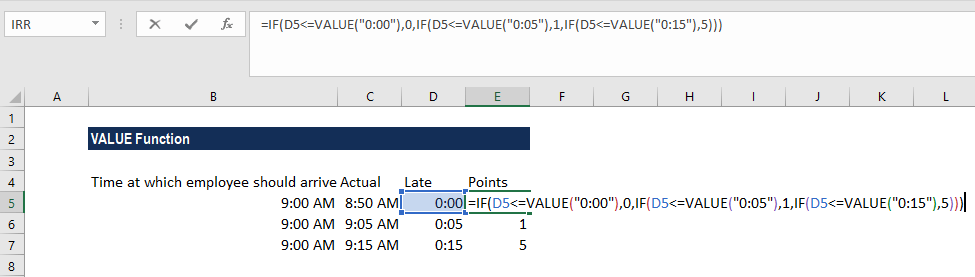We get the results below: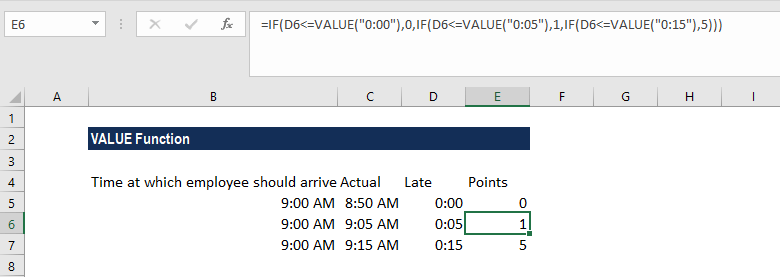The formula first checks the tardiness by time in D5 to see if it’s 0, then zero points are assigned. If the result of the logical test above is FALSE, the formula checks to see if C3 is less than the next threshold, which is 5 minutes. It will then assign 1.

The same pattern repeats at each threshold. As the tests are run in order, from smallest to largest, there is no need for more complicated bracketing.

The VALUE function is used to make Excel treat the time value at each threshold as a number instead of next.

### Things to remember about the VALUE Function

1. As per Microsoft, the VALUE function is used for compatibility with other spreadsheet programs.
2. The function converts text that appears in a recognized format (a number, date, or time format) into a numeric value.

Thanks for reading CFI’s guide to this important Excel function. By taking the time to learn and master these functions, you’ll significantly speed up your financial analysis. To learn more, check out these additional CFI resources:

• Excel Functions for Finance